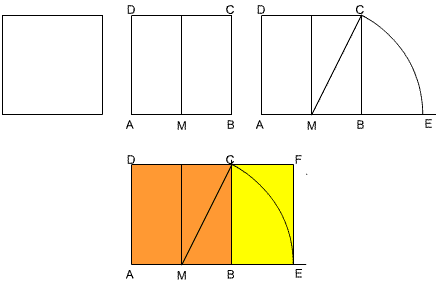#### You may also like### Kissing

Two perpendicular lines are tangential to two identical circles that touch. What is the largest circle that can be placed in between the two lines and the two circles and how would you construct it?### Gold Again

Without using a calculator, computer or tables find the exact values of cos36cos72 and also cos36 - cos72.### Pentabuild

Explain how to construct a regular pentagon accurately using a straight edge and compass.

# Golden Construction

##### Age 16 to 18Challenge Level

In this problem you start with a square, construct a golden rectangle and calculate the value of the golden ratio.

When you cut a square off a golden rectangle you are left with another rectangle whose sides are in the same ratio. In the diagram below, if you remove the orange square from rectangle $AEFD$, you are left with the yellow rectangle whose sides are in the same ratio as $AEFD$.(1) Follow the instructions for drawing the rectangle $AEFD$. You can make the most accurate drawing by using a ruler and compasses. Draw a square $ABCD$ of side length 10 cm. Bisect $AB$ at $M$ and draw an arc of radius $MC$ to meet $AB$ produced at $E$. If you prefer you can just measure $MC$ and mark $E$ on $AB$ so that $ME=MC$. Draw $EF$ perpendicular to $AB$ to meet $DC$ produced at $F$.

Measure $AE$ and $BE$. From your measurements calculate the ratios $AE$/$AD$ and $BC/BE$. What do you notice?

(2) Calculate the exact lengths of $MC$, $AE$ and $BE$. Calculate the exact value of the ratios $AE$/$AD$ and $BC$/$BE$ and prove that they are equal. [Note: To get exact values you must work with surds and you will not be able to use a calculator.]

(3) Suppose this ratio is denoted by $\phi$ and take 10 cm as 1 unit then $AE$ is $\phi$ units. Show that $BE$ is $1/\phi$ units and hence $$\phi = 1 + {1\over \phi}\quad (1)$$

Explain just from equation (1), and without solving the equation, why the equation must have a solution between 1 and 2.

(4) Draw the graphs of $y=x$, $y=1/x$ and $y=1+1/x$ on the same axes and use your graph to find an approximate value for $\phi$.

(5) Solve equation (1) to find the value of $\phi$.